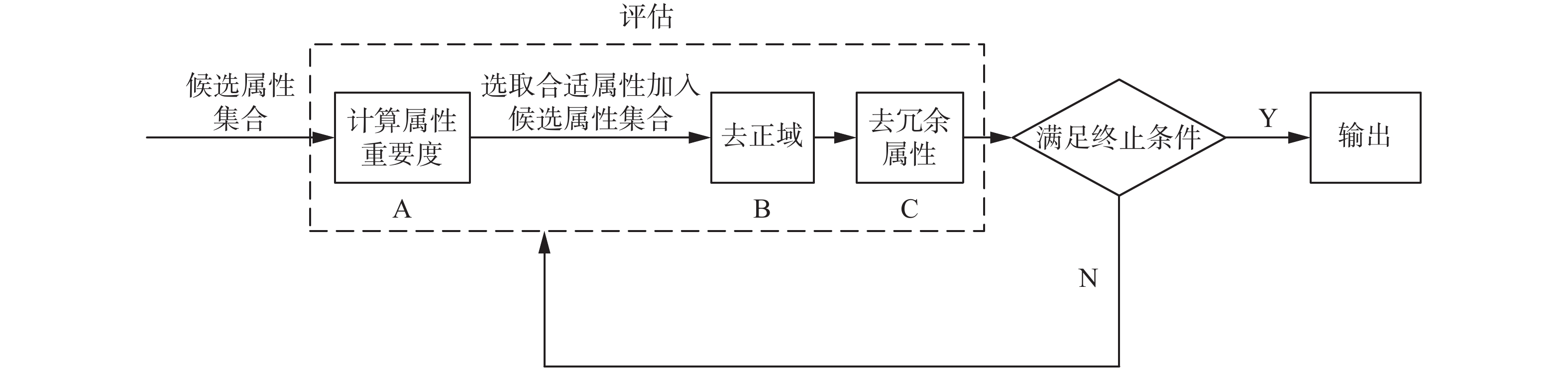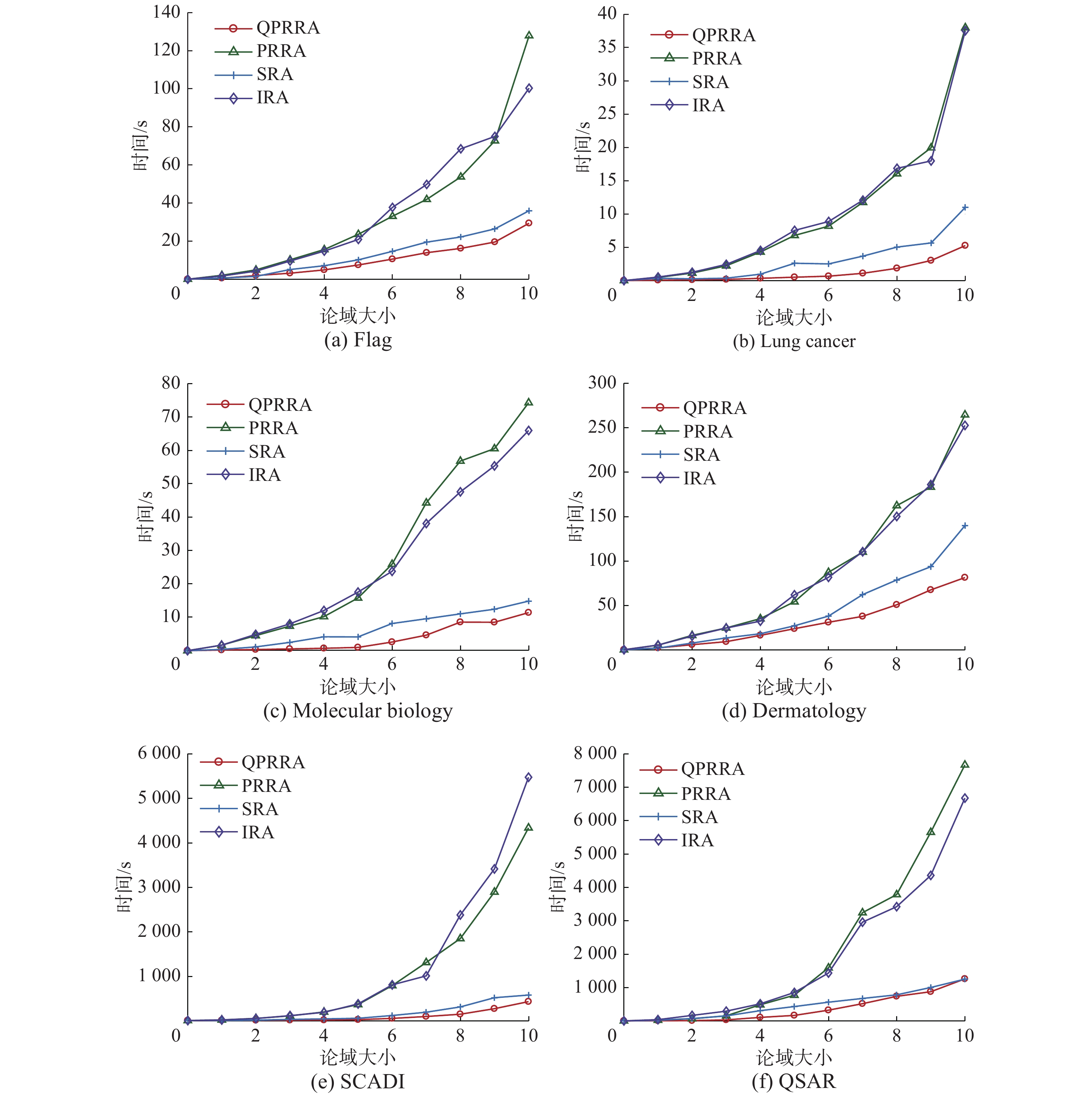﻿ 集值信息系统的快速正域约简
«上一篇文章快速检索 高级检索

 智能系统学报2019, Vol. 14Issue (3): 471-478  DOI: 10.11992/tis.2018040590

### 引用本文CHEN Manru, ZHANG Nan, TONG Xiangrong, et al. Quick positive region reduction in set-valued information systems[J]. CAAI Transactions on Intelligent Systems, 2019, 14(3): 471-478. DOI: 10.11992/tis.201804059.### 文章历史

1. 烟台大学 数据科学与智能技术山东省高校重点实验室，山东 烟台 264005;
2. 上海大学 计算机工程与科学学院，上海 200444

Quick positive region reduction in set-valued information systems
CHEN Manru 1, ZHANG Nan 1, TONG Xiangrong 1, YUE Xiaodong 2
1. Key Lab for Data Science and Intelligence Technology of Shandong Higher Education Institutes, Yantai University, Yantai 264005, China;
2. School of Computer Engineering and Science, Shanghai University, Shanghai 200444, China
Abstract: This study aims to propose a quick positive reduction algorithm based on the heuristic method to increase the efficiency of the set-valued positive reduction algorithm under large-scale data. The definitions of attribute independence and attribute importance isotonicity are introduced in the set-valued information system by investigating the influence of an attribute and object on the efficiency of algorithm during the reduction process, and the relevant theorem, fast algorithm, and practical example for improving the efficiency of the algorithm are introduced. Finally, the experimental results show the efficiency and effectiveness of the proposed method and its better efficiency in comparison to that of the original algorithm.
Key words: attribute reduction    rough set    set-valued information systems    feature selection    heuristic algorithm    positive region reduction    quick algorithm reduction    rough approximations

Skowron等认为差别矩阵方法虽然可以求得数据集的所有约简，但是其效率相对于启发式算法较低，故应用性较差。由于现实生活中数据信息量的不断增大，集值系统中属性约简算法的效率需要做进一步研究。罗川等在集值序决策系统中通过计算粗糙近似提出了基于增加和删除策略的增量式算法；Zhang等在集值系统中提出了构造关系矩阵的基本方法并且通过研究属性集变化时关系矩阵变化的性质，得到了关系矩阵更新的增量式方法；刘莹莹等在集值信息系统中通过定义一种新的相似度，提出基于相似度的启发式算法；马建敏等在集值信息系统中引入信息量等概念，提出一种新的启发式约简算法。

1 基础知识 1.1 集值信息系统相关基础知识表 1 集值决策系统 Tab.1 Set-valued decision systems

 ${{{T}}_A} = \{ (x, y) \in U \times U|\forall a \in A, a(x) \cap a(y) \ne \varnothing \}\text{。}$

X 的下近似是在相容关系 A 下确定属于 X 的全体对象集合，而 X 的上近似是在相容关系 A 下可能属于 X 的全体对象集合。

1.2 集值信息系统的正域约简算法

 ${\gamma _A}(D) = \frac{{|{\rm{POS}}_A(D)|}}{{|U|}}$

1) ${\gamma _A}(D) = {\gamma _C}(D)$

2) $\forall B \subset A$ ${\gamma _B}(D) < {\gamma _A}(D)$

 ${\rm{Sig}}^{{\rm{inner}}}(a, A, C, D, U) = {\gamma _A}(D) - {\gamma _{A - \{ a\} }}(D)$

 ${\rm{Sig}}^{{\rm{outer}}}(a, A, C{\rm{, }}D, U) = {\gamma _{A \cup \{ a\} }}(D) - {\gamma _A}(D)$

1) $R = \varnothing$

2)计算 ${\rm{Sig}}^{{\rm{inner}}}({a_i}, C, C, D, U)$ $i \in {\rm{\{ 1, 2, }} \cdots , |C|{\rm{\} }}$ ，如果 ${\rm{Sig}}^{{\rm{inner}}}({a_i}, C, C, D, U) > 0$ ，则将 ${a_i}$ 存入 R 中。

3)若 ${\gamma _R}(D) = {\gamma _C}(D)$ ，则终止；否则对条件属性 $C - R$ 进行如下操作：

4)对 R 中的每个条件属性 ${a_i}$ 进行如下操作：

①计算 ${\gamma _{R - \{ {a_i}\} }}(D)$

②若 ${\gamma _{R - \{ {a_i}\} }}(D) \!=\! {\gamma _C}(D)$ ，则 ${a_i}$ 为冗余属性， $R \!=\! R \!-\! \{ {a_i}\}$ ，否则 ${a_i}$ 为非冗余属性，R 保持不变。

5)返回 R

1)表1中经求其核属性集为 $R = \{ {a_3}, {a_4}\}$ ，决策属性D相对于条件属性C的近似分类质量为 ${\gamma _C}(D) = 0.625$

2)对 $C - R$ 中每个条件属性重复进行如下操作：

3)去除集合 R 中的冗余属性，由于 ${\gamma _{R - \{ {a_1}\} }}(D) \ne$ ${\gamma _C}(D)$ ，故集合 R 中无冗余属性，算法终止。

2 集值系统的快速正域约简

Qian等[21-22]提出了正向近似的加速原理。本节在文献[21-22]算法的基础上介绍集值信息系统下的正域属性约简算法的加速原理以及相关性质，给出了算法的伪代码描述和两个算法的时间复杂度对比与分析。

 ${\rm{POS}}_{{P_{i + 1}}}(U, D) = {\rm{PO}}{{\rm{S}}_{{P_i}}}(U, D) \cup {\rm{PO}}{{\rm{S}}_{{P_{i + 1}}}}({U_{i + 1}}, D)\text{。}$

 $\begin{array}{c}\displaystyle\frac{{{\rm{Si}}{{\rm{g}}^{{\rm{outer}}}}(a, A, C, D, U)}}{{{\rm{Si}}{{\rm{g}}^{{\rm{outer}}}}(a, A, C', D, {U^ * })}}= \displaystyle\frac{{\gamma _{A \cup \{ a\} }^{(C, U)}(D) - \gamma _A^{(C, U)}(D)}}{{\gamma _{A \cup \{ a\} }^{(C', {U^ * })}(D) - \gamma _A^{(C', {U^ * })}(D)}} = \\ \displaystyle\frac{{{\rm{|}}{U^ * }{\rm{|}}}}{{{\rm{|}}U{\rm{|}}}}\frac{{{\rm{|POS}}_{A \cup \{ a\} }^C(U, D){\rm{|}} - {\rm{|POS}}_A^C(U, D){\rm{|}}}}{{{\rm{|POS}}_{A \cup \{ a\} }^{C'}({U^ * }, D){\rm{|}} - {\rm{|POS}}_A^{C'}({U^ * }, D){\rm{|}}}} = \\ \displaystyle\frac{{{\rm{|}}{U^ * }{\rm{|}}}}{{{\rm{|}}U{\rm{|}}}}\frac{{{\rm{|POS}}_{A \cup \{ a\} }^C(U, D){\rm{|}} - {\rm{|POS}}_A^C(U, D){\rm{|}}}}{{{\rm{|POS}}_{A \cup \{ a\} }^C({U^ * }, D){\rm{|}} - {\rm{|POS}}_A^C({U^ * }, D){\rm{|}}}} = \\ \displaystyle\frac{{{\rm{|}}{U^ * }{\rm{|}}}}{{{\rm{|}}U{\rm{|}}}}\frac{{{\rm{|POS}}_{A \cup \{ a\} }^C(U, D){\rm{|}} - {\rm{|POS}}_A^C(U, D){\rm{|}}}}{{{\rm{|POS}}_{A \cup \{ a\} }^C(U, D){\rm{|}} - {\rm{|POS}}_A^C(U, D){\rm{|}}}} = \displaystyle\frac{{{\rm{|}}{U^ * }{\rm{|}}}}{{{\rm{|}}U{\rm{|}}}}\end{array}$

1)令 $i = 1$ ${R_1} = R$ ${U_1} = U$ ${C_1} = C$ ${C_d} = \varnothing$ $R =\varnothing$

2)对条件属性 ${C_i} - R$ 进行如下操作：计算 ${\rm{Si}}{{\rm{g}}^{{\rm{outer}}}}$ $({a_0}, R, {C_i}, D, {U_i}) \!\!=\!\! \max \{ {\rm{Si}}{{\rm{g}}^{{\rm{outer}}}}({a_k}, R, {C_i},D, {U_i})\}$ $R \leftarrow$ $R \cup \{ {a_0}\}$ ，其中 ${a_k} \in {C_i} - R$ 。若有多个最大值，则选择与 R 组合数最少的属性。

3)计算 ${U_{i + 1}} = {U_i} - {\rm{POS}}_R^{{C_i}}({U_i}, D)$

4)计算 $C_d^{{U_i}} = \{ a \in {C_i} - R:U/{{{T}}_R} = U/{{{T}}_{R \cup \{ a\} }}\}$ ${C_{i + 1}} =$ ${C_i} - C_d^{{U_i}}$ ，令 $i = i + 1$

5)若 $\gamma _R^{{U_i}}(D) = \gamma _{{C_i}}^{{U_i}}(D)$ ，转到6)，否则转到2)。

6)对 $C - R$ 中的每个条件属性 ${a_k}$ ，进行如下操作：

①计算 ${\gamma _{R - \{ {a_k}\} }}(D)$

②若 ${\gamma _{R - \{ {a_k}\} }}(D) = {\gamma _C}(D)$ ，则 ${a_k}$ 为冗余属性，R = $R - \{ {a_k}\}$ ，否则 ${a_k}$ 为非冗余属性，R 保持不变。

7)返回RDownload: 图 1 算法2原理流程 Fig. 1 Flow chart for algorithm 2Download: 图 2 算法PRRA和算法QPRRA计算时间 Fig. 2 Time reguired for PRRA and QPRRA versus the data size

3 实验分析表 2 数据集描述 Tab.2 Description of data sets表 3 算法PRRA和QPRRA的计算时间和约简结果 Tab.3 Time regurired and attribute reduction of the algorithms表 4 算法PRRA和QPRRA在Lung Cancer数据集上每轮迭代对象和属性的变化 Tab.4 Changes of the number of objects and attributes within each loop of algorithms PRRA and QPRRA on Lung Cancer
4 结束语

  PAWLAK Z. Rough sets[J]. International journal of computer and information sciences, 1982, 11(5): 341-356. DOI:10.1007/BF01001956 (0)  王国胤, 姚一豫, 于洪. 粗糙集理论与应用研究综述[J]. 计算机学报, 2009, 32(7): 1229-1246. WANG Guoyin, YAO Yiyu, YU Hong. A survey on rough set theory and applications[J]. Chinese journal of computers, 2009, 32(7): 1229-1246. (0)  MIAO Duoqian, ZHAO Yan, YAO Yiyu, et al. Relative reducts in consistent and inconsistent decision tables of the Pawlak rough set model[J]. Information sciences, 2009, 179(24): 4140-4150. DOI:10.1016/j.ins.2009.08.020 (0)  LI Hua, LI Deyu, ZHAI Yanhui, et al. A novel attribute reduction approach for multi-label data based on rough set theory[J]. Information sciences, 2016, 367/368: 827-847. DOI:10.1016/j.ins.2016.07.008 (0)  YAO Yiyu, ZHAO Yan. Attribute reduction in decision-theoretic rough set models[J]. Information sciences, 2008, 178(17): 3356-3373. DOI:10.1016/j.ins.2008.05.010 (0)  JIA Xiuyi, SHANG Lin, ZHOU Bin, et al. Generalized attribute reduct in rough set theory[J]. Knowledge-based systems, 2016, 91: 204-218. DOI:10.1016/j.knosys.2015.05.017 (0)  张楠, 苗夺谦, 岳晓冬. 区间值信息系统的知识约简[J]. 计算机研究与发展, 2010, 47(8): 1362-1371. ZHANG Nan, MIAO Duoqian, YUE Xiaodong. Approaches to knowledge reduction in interval-valued information systems[J]. Journal of computer research and development, 2010, 47(8): 1362-1371. (0)  HU Qinghua, ZHAO Hui, XIE Zongxia, et al. Consistency based attribute reduction[C]//Advances in Knowledge Discovery and Data Mining. Berlin, Heidelberg, Germany, 2007: 96–107. (0)  GUAN Yanyong, WANG Hongkai. Set-valued information systems[J]. Information sciences, 2006, 176(17): 2507-2525. DOI:10.1016/j.ins.2005.12.007 (0)  QIAN Yuhua, DANG Chuanyin, LIANG Jiye, et al. Set-valued ordered information systems[J]. Information sciences, 2009, 179(16): 2809-2832. DOI:10.1016/j.ins.2009.04.007 (0)  杨习贝, 张再跃, 张明. 集值信息系统中的模糊优势关系粗糙集[J]. 计算机科学, 2011, 38(2): 234-237. YANG Xibei, ZHANG Zaiyue, ZHANG Ming. Fuzzy dominance-based rough set in set-valued information system[J]. Computer science, 2011, 38(2): 234-237. DOI:10.3969/j.issn.1002-137X.2011.02.055 (0)  HUANG Yanyong, LI Tianrui, LUO Chuan, et al. Dynamic variable precision rough set approach for probabilistic set-valued information systems[J]. Knowledge-based systems, 2017, 122: 131-147. DOI:10.1016/j.knosys.2017.02.002 (0)  WEI Wei, CUI Junbiao, LIANG Jiye, et al. Fuzzy rough approximations for set-valued data[J]. Information sciences, 2016, 360: 181-201. DOI:10.1016/j.ins.2016.04.005 (0)  ZHANG Hongying, YANG Shuyun. Feature selection and approximate reasoning of large-scale set-valued decision tables based on α-dominance-based quantitative rough sets [J]. Information sciences, 2017, 378: 328-347. DOI:10.1016/j.ins.2016.06.028 (0)  SKOWRON A, RAUSZER C. The discernibility matrices and functions in information systems[C]//Intelligent Decision Support Theory and Decision Library. Dordrecht, Netherlands, 1992: 331–362. (0)  LUO Chuan, LI Tianrui, CHEN Hongmei, et al. Fast algorithms for computing rough approximations in set-valued decision systems while updating criteria values[J]. Information sciences, 2015, 299: 221-242. DOI:10.1016/j.ins.2014.12.029 (0)  ZHANG Junbo, LI Tianrui, RUAN Da, et al. Rough sets based matrix approaches with dynamic attribute variation in set-valued information systems[J]. International journal of approximate reasoning, 2012, 53(4): 620-635. DOI:10.1016/j.ijar.2012.01.001 (0)  刘莹莹, 吕跃进. 基于相似度的集值信息系统属性约简算法[J]. 南京大学学报 (自然科学版), 2015, 51(2): 384-389. LIU Yingying, LYU Yuejin. Attribute reduction in set-valued information system based on similarity[J]. Journal of Nanjing university (natural sciences), 2015, 51(2): 384-389. (0)  马建敏, 张文修. 基于信息量的集值信息系统的属性约简[J]. 模糊系统与数学, 2013, 27(2): 177-182. MA Jianmin, ZHANG Wenxiu. Information quantity-based attribute reduction in set-valued information systems[J]. Fuzzy systems and mathematics, 2013, 27(2): 177-182. DOI:10.3969/j.issn.1001-7402.2013.02.028 (0)  苗夺谦, 李道国. 粗糙集理论、算法与应用[M]. 北京: 清华大学出版社, 2008. (0)  QIAN Yuhua, LIANG Jiye, PEDRYCZ W, et al. Positive approximation: an accelerator for attribute reduction in rough set theory[J]. Artificial intelligence, 2010, 174(9/10): 597-618. (0)  钱宇华, 梁吉业, 王锋. 面向非完备决策表的正向近似特征选择加速算法[J]. 计算机学报, 2011, 34(3): 435-442. QIAN Yuhua, LIANG Jiye, WANG Feng. A positive-approximation based accelerated algorithm to feature selection from incomplete decision tables[J]. Chinese journal of computers, 2011, 34(3): 435-442. (0)